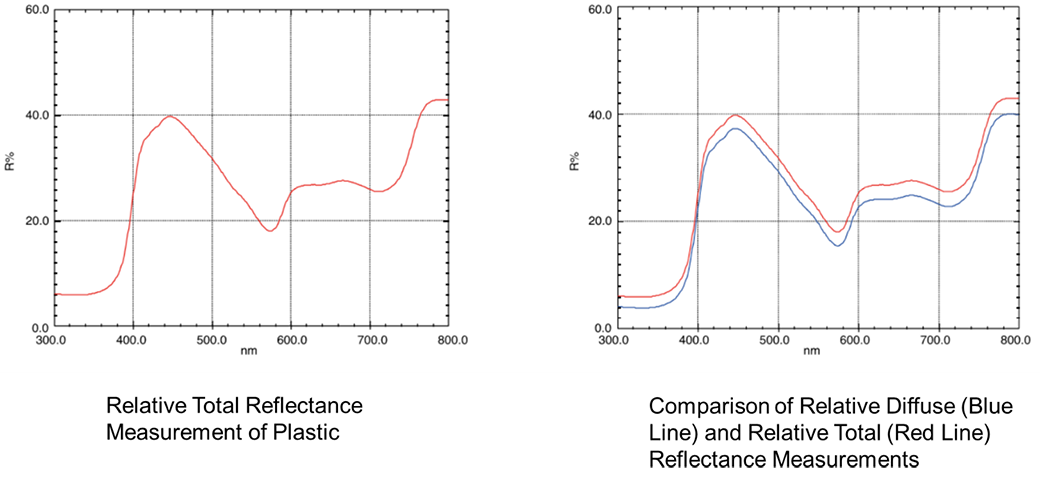# An example of total reflectance measurementThe graphs here show the results from using a barium sulfate reference plate to measure the relative total reflectance of an opaque plastic material. Relative Diffuse Reflectance Measurement. The graph at right shows a comparison of the relative diffuse reflectance spectrum and relative total reflectance spectrum. Because the relative total reflectance includes the specular reflectance components, the reflectance level is higher than the relative diffuse reflectance. The difference between is the approximate specular contribution.

Back to Index